# Ahlswede-Daykin inequality

four-functions inequality

An inequality in which an inequality for four functions on a finite distributive lattice applies also to additive extensions of the functions on lattice subsets. Let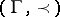be a finite distributive lattice (see also FKG inequality), such as the power set of a finite set ordered by proper inclusion. For subsets,of, defineand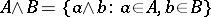. Iforis empty,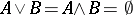. Given, let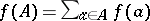.

The Ahlswede–Daykin inequality says that if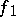,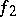,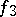, andmapinto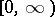such thatthen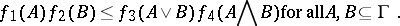See [a1] or [a2], [a4], [a7] for a proof.

The inequality is very basic and is used in proofs of other inequalities (cf. [a2], [a3], [a4], [a5], [a7]), including the FKG inequality [a6] and the Fishburn–Shepp inequality [a3], [a8].